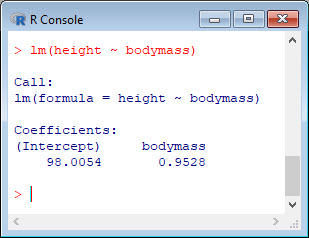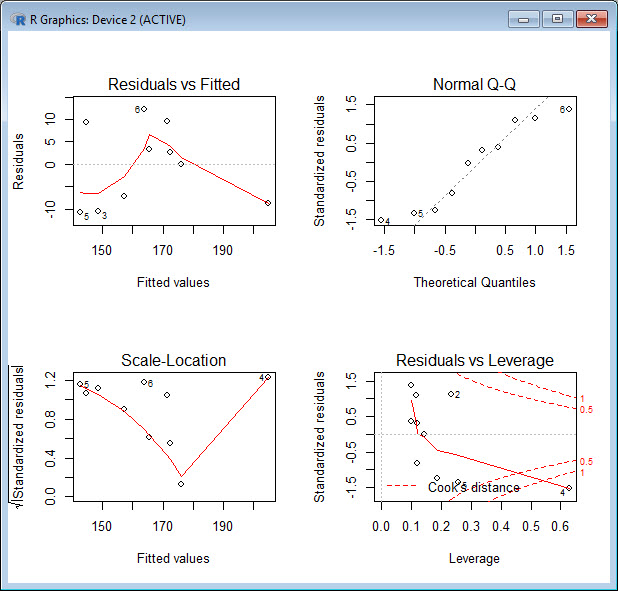Last time we created two variables and used the `lm()` command to perform a least squares regression on them, treating one of them as the dependent variable and the other as the independent variable. Here they are again.

```height = c(176, 154, 138, 196, 132, 176, 181, 169, 150, 175) bodymass = c(82, 49, 53, 112, 47, 69, 77, 71, 62, 78)```

Today we learn how to obtain more useful diagnostic information about a regression model. As before, we perform the regression.
`lm(height ~ bodymass)`Now we can use several R diagnostic plots and influence statistics to understand our model. These diagnostic plots are as follows:

1. Residuals vs. fitted values
2. Q-Q plots
3. Scale Location plots
4. Cook’s distance plots.

To use R’s regression diagnostic plots, we set up the regression model as an object and create a plotting environment of two rows and two columns. Then we use the `plot()` command, treating the model as an argument.
`model <- lm(height ~ bodymass)`
`par(mfrow = c(2,2))`
`plot(model)`The first plot gives the residuals plotted against the fitted values. If our data had no scatter, so that all points fell on the regression line, then every datum would fall on the horizontal line of the first plot. The red curve is a smoothed representation of the residuals, and ideally should be relatively flat and close to the horizontal line; that is – there should be no trend. However, this is not the case for our data. To summarise: the first plot (residuals vs. fitted values) should look more or less random, but that is not what we see here.

The second plot is a Quantile-Quantile (Q-Q) plot of the residuals. This plot helps us to identify whether or not the residuals are distributed normally. In our example, most of the points lie close to the dashed line. If the residuals were distributed normally, all of the points would lie along this line. For real data, there will be deviations, but any deviations should be small. To summarise: the second plot (normal Q-Q errors) will give a straight line if the errors are distributed normally, but points 4, 5 and 6 deviate from the straight line.

The third plot (Scale Location) gives the fitted values, plotted against the square root of the standardized residuals (giving a mean of zero and a variance of unity). Large residuals (both positive and negative) appear at the top of the plot, while small residuals appear at the bottom. The red curve indicates any trend in the standardised residuals. If the red line is reasonably flat, then the variance in the residuals doesn’t change greatly over the range of the independent variable (and we have homoscedastic data). To summarise: the third plot is similar to the first, and should look random. However, ours does not.

The last plot (lower right) gives the standardized residuals, plotted against leverage. For normally distributed residuals, the standardized residuals will be centred symmetrically on zero. Leverage provides one measure of the extent to which each point influences the regression. Because the regression line passes through the geometric centre of the data, points that lie far from the geometric centre have greater leverage, and their leverage increases when the points are relatively isolated (i.e. there are not many points close to the point of interest). The leverage of any point depends on the distance from the geometric centre and on the isolation of that point. Data that are simultaneously outliers and have high leverage influence both the slopes and intercept of a regression model. We see that point 4 has high leverage.

The last plot also gives contours of Cook’s distance, which is a measure of how much the regression would change if a point were omitted from the regression. Cook’s distance increases when leverage is large. When the residuals are large, any point far from the geometric centre and that has a large residual distorts the regression. Ideally, the red smoothed line remains close to the horizontal dashed line and ideally no points have a large Cook’s distance (i.e. > 0.5). Neither of these two conditions apply for our data. To summarise: the last plot (Cook’s distance) tells us which points have the greatest influence on the regression (leverage points). We see that point 4 (having both high leverage and high Cook’s Distance) has considerable influence on the model.

David

#### Annex: R codes used

```# Create two variables.
height = c(176, 154, 138, 196, 132, 176, 181, 169, 150, 175)
bodymass = c(82, 49, 53, 112, 47, 69, 77, 71, 62, 78)

# Estimate the regression model.
lm(height ~ bodymass)

# Store the regression model as an object.
model <- lm(height ~ bodymass)

# Create a plotting environment of two rows and two columns and plot the model.
par(mfrow = c(2,2))
plot(model)
```